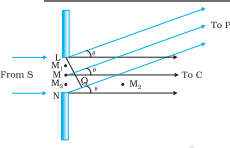# (a) Derive the relation   for the first minimum of the diffraction pattern produced due to a single slit of width ‘a’ using light of wavelength  .(b) State with reason, how the linear width of central maximum will be affected if(i) monochromatic yellow light is replaced with red light, and(ii) distance between the slit and the screen is increased.(c) Using the monochromatic light of same wavelength in the experimental set-up of the diffraction pattern as well as in the interference pattern where the slit separation is 1 mm, 10 interference fringes are found to be within the central maximum of the diffraction pattern. Determine the width of the single slit, if the screen is kept at the same distance from the slit in the two cases.

(a)

from the diagram the path difference between the waves from L and N

Where 'a' is the slit separationThe path difference = when the first minimum is obtained at P (Imagine the slit be divided into two halves, for each wavelet from the first half of the slit has a corresponding wavelet from the second half of slit differing by a path of  and here cancel each other)

Hence, the condition for the first minimum would be  :-

(b)

The linear width of central maxima

(i) As we know that wave length  of red light is more than yellow light.

So,

Hence, the linear width of the central maximum will increase if monochromatic yellow light is replaced with the red light.

(ii) If the distance between the slit and screen (d) is increased then also the linear width of the central maximum will increase.

(c) For the diffraction pattern;

d=slit separation

and

N=10 fringes (given)

So,

The wavelength  and  is same for both patterns.

Since, the linear width of the central maximum of single is given by,

where a=width of slit.

y=x(given)

10 fringes are found within central maxima

Thus, the width of the single slit

### Preparation Products

##### Knockout KCET 2021

An exhaustive E-learning program for the complete preparation of KCET exam..

₹ 4999/- ₹ 2999/-
##### Knockout KCET JEE Main 2021

It is an exhaustive preparation module made exclusively for cracking JEE & KCET.

₹ 27999/- ₹ 16999/-
##### Knockout NEET Sept 2020

An exhaustive E-learning program for the complete preparation of NEET..

₹ 15999/- ₹ 6999/-
##### Rank Booster NEET 2020

This course will help student to be better prepared and study in the right direction for NEET..

₹ 9999/- ₹ 4999/-